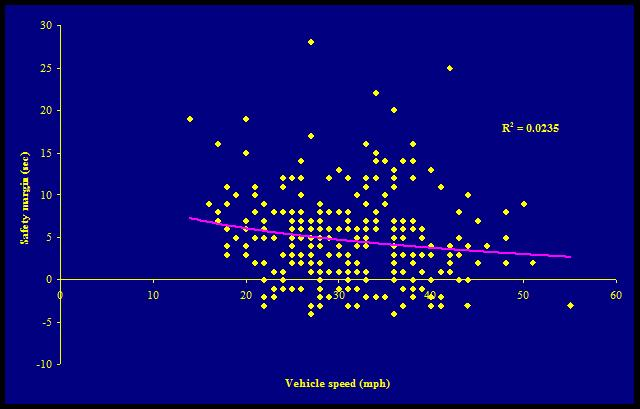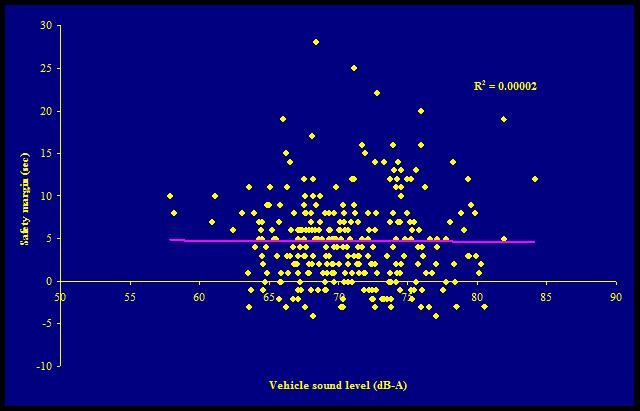Figure 3.
Effect of vehicle speed
[Note: Safety margin of 0 = detection time of 7 seconds]Description

(NOTE: this description is for the figure in the article, the figure on this page is the same except that the time of detection is indicated in terms of safety margin, with a safety margin of 0 being a detection time of 7 seconds.):

The figure is a scatterplot.
The x-axis is labeled "Vehicle speed (mph)" and has 7 values, from left to right: 0, 10, 20, 30, 40, 50, and 60.
The y-axis is labeled "Detection time (sec)" and has 9 values, from bottom to top: 0, 5, 10, 15, 20, 25, 30, 35, and 40.
A bar runs through the graph from about 15 miles per hour to 55 miles per hour with a range of 10–15 seconds detection time.
Black diamonds are plotted heavily between 4 and 5 seconds detection time and between 17 and 51 mph, and scattered sporadically between 5 and 21 seconds of detection time and 15 and 42 mph; one outlier is at 55 mph and 4 seconds of detection time.

Table:
Effect of vehicle sound level
[Note: Safety margin of 0 = detection time of 7 seconds]Description:

The figure is a scatterplot.
The x-axis is labeled "Vehicle sound level (dB-A)" and has 9 values, from left to right: 50, 55, 60, 65, 70, 75, 80, 85, and 90.
The y-axis is labeled "Safety margin (sec)" and has 9 values, from bottom to top: -10, -5, 0, 5, 10, 15, 20, 25 and 30.
A bar runs through the graph horizontally at about 4.5 seconds of safety margin from about 57 to 85 dB-A.
Black diamonds are plotted heavily between -4 and 11 seconds safety margin and between 63 and 80 dB-A.
The 2 quietest vehicles are about 58 dB-A and their safety margins are 8 and 10 seconds, the next 2 quietest vehicles are about 62 dB-A with safety margins of about 7 and 10 seconds.
There are also sparsely scattered diamonds plotted between 11 and 28 seconds and between 65 and 85 dB-A.